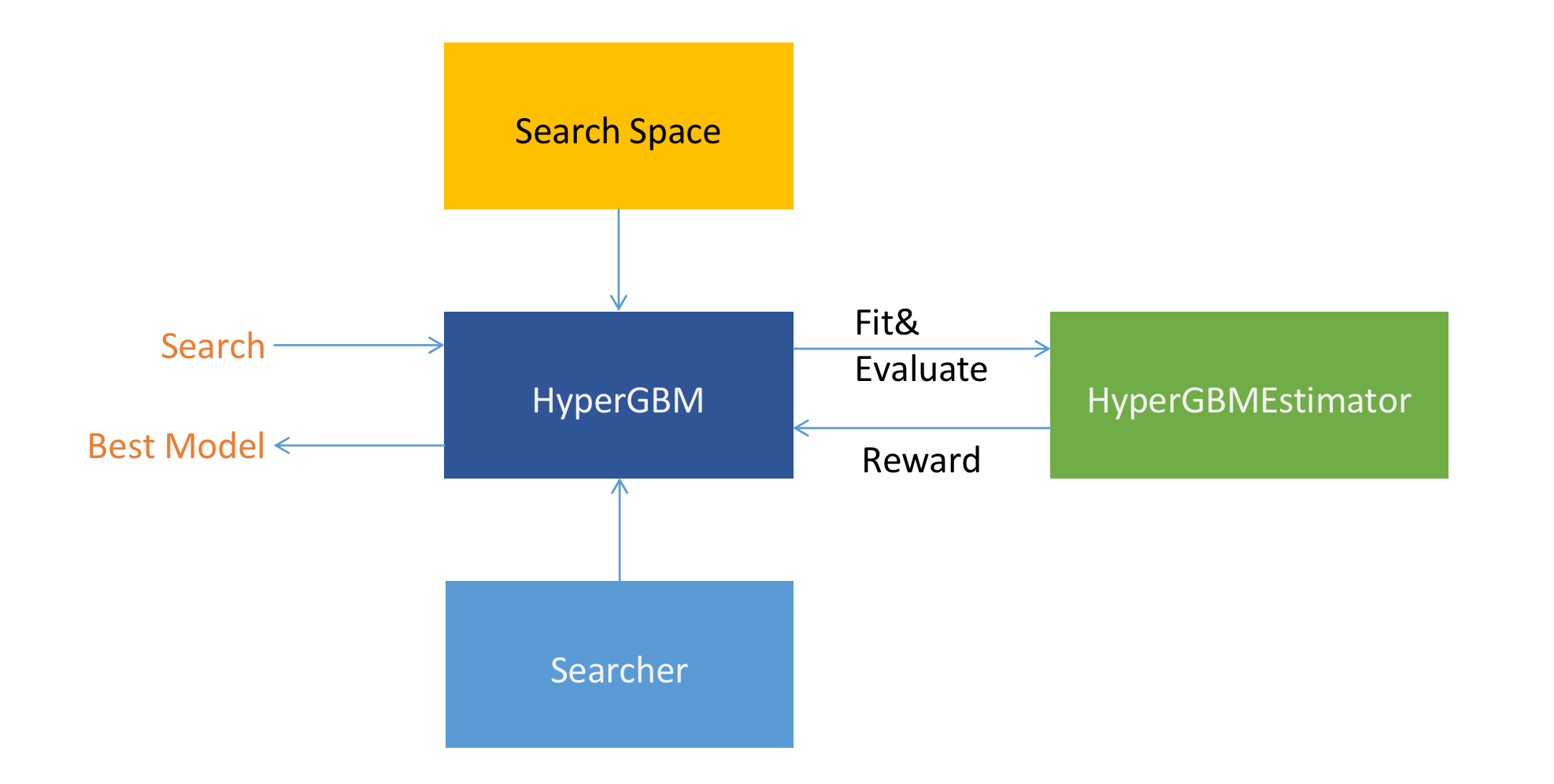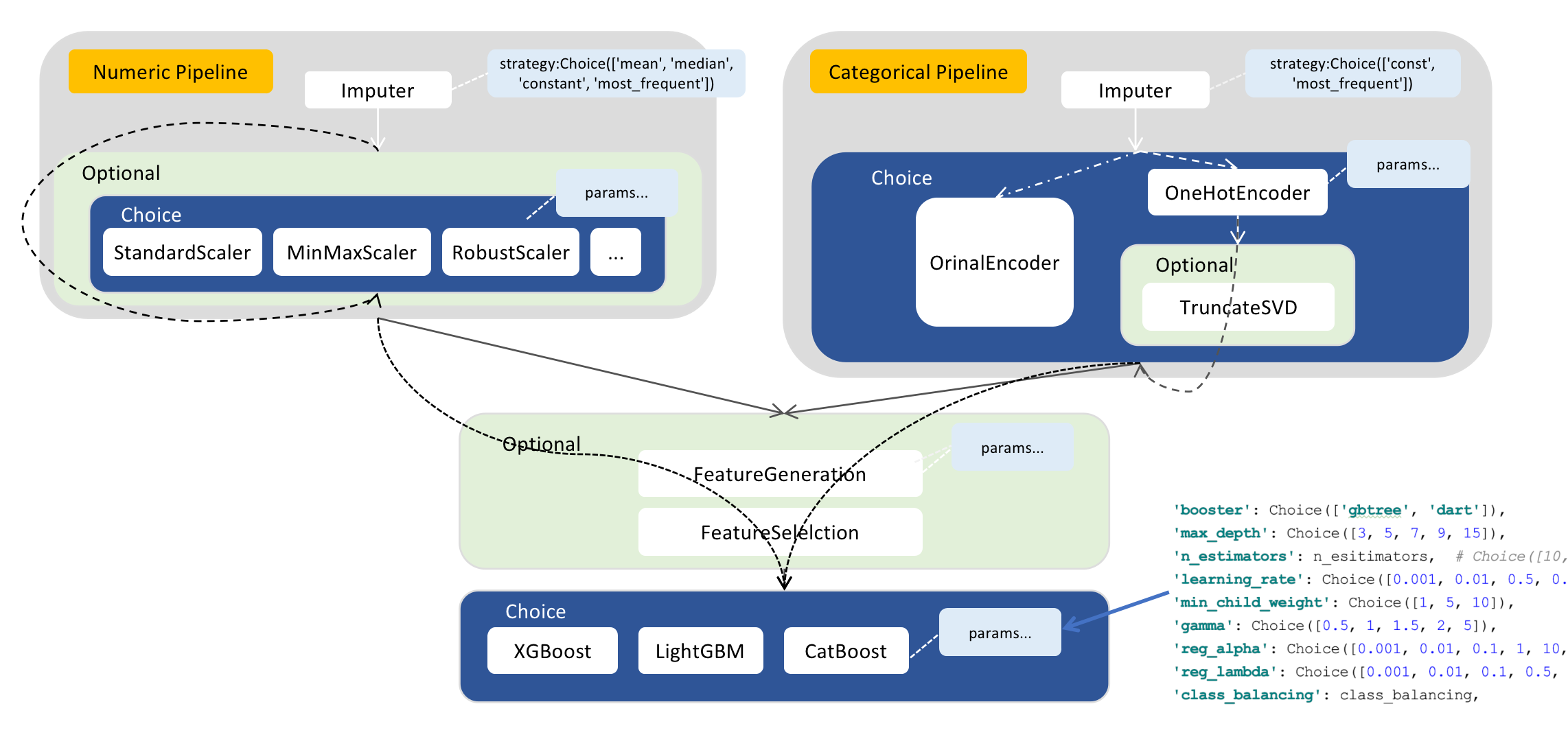# Overview¶

## What is HyperGBM¶

HyperGBM is a library that supports full-pipeline AutoML, which completely covers the end-to-end stages of data cleaning, preprocessing, feature generation and selection, model selection and hyperparameter optimization.It is a real-AutoML tool for tabular data.

Unlike most AutoML approaches that focus on tackling the hyperparameter optimization problem of machine learning algorithms, HyperGBM can put the entire process from data cleaning to algorithm selection in one search space for optimization. End-to-end pipeline optimization is more like a sequential decision process, thereby HyperGBM uses reinforcement learning, Monte Carlo Tree Search, evolution algorithm combined with a meta-learner to efficiently solve such problems. As the name implies, the ML algorithms used in HyperGBM are all GBM models, and more precisely the gradient boosting tree model, which currently includes XGBoost, LightGBM and Catboost. The underlying search space representation and search algorithm in HyperGBM are powered by the Hypernets project a general AutoML framework.

## Main components¶

In this section, we briefly cover the main components in HyperGBM. As shown below:• HyperGBM(HyperModel)

HyperGBM is a specific implementation of HyperModel (for HyperModel, please refer to the Hypernets project). It is the core interface of the HyperGBM project. By calling the `search` method to explore and return the best model in the specified `Search Space` with the specified `Searcher`.

• Search Space

Search spaces are constructed by arranging ModelSpace(transformer and estimator), ConnectionSpace(pipeline) and ParameterSpace(hyperparameter). The transformers are chained together by pipelines while the pipelines can be nested. The last node of a search space must be an estimator. Each transformer and estimator can define a set of hyperparameterss.The code example of Numeric Pipeline is as follows：

```import numpy as np
from hypergbm.pipeline import Pipeline
from hypergbm.sklearn.transformers import SimpleImputer, StandardScaler, MinMaxScaler, MaxAbsScaler, RobustScaler, LogStandardScaler
from hypernets.core.ops import ModuleChoice, Optional, Choice
from tabular_toolbox.column_selector import  column_number_exclude_timedelta

def numeric_pipeline_complex(impute_strategy=None, seq_no=0):
if impute_strategy is None:
impute_strategy = Choice(['mean', 'median', 'constant', 'most_frequent'])
elif isinstance(impute_strategy, list):
impute_strategy = Choice(impute_strategy)

imputer = SimpleImputer(missing_values=np.nan, strategy=impute_strategy, name=f'numeric_imputer_{seq_no}',
force_output_as_float=True)
scaler_options = ModuleChoice(
[
LogStandardScaler(name=f'numeric_log_standard_scaler_{seq_no}'),
StandardScaler(name=f'numeric_standard_scaler_{seq_no}'),
MinMaxScaler(name=f'numeric_minmax_scaler_{seq_no}'),
MaxAbsScaler(name=f'numeric_maxabs_scaler_{seq_no}'),
RobustScaler(name=f'numeric_robust_scaler_{seq_no}')
], name=f'numeric_or_scaler_{seq_no}'
)
pipeline = Pipeline([imputer, scaler_optional],
name=f'numeric_pipeline_complex_{seq_no}',
columns=column_number_exclude_timedelta)
return pipeline
```
• Searcher

Searcher is an algorithm used to explore a search space.It encompasses the classical exploration-exploitation trade-off since, on the one hand, it is desirable to find well-performing model quickly, while on the other hand, premature convergence to a region of suboptimal solutions should be avoided. Three algorithms are provided in HyperGBM: MCTSSearcher (Monte-Carlo tree search), EvolutionarySearcher and RandomSearcher.

• HyperGBMEstimator

HyperGBMEstimator is an object built from a sample in the search space, including the full preprocessing pipeline and a GBM model. It can be used to `fit` on training data, `evaluate` on evaluation data, and `predict` on new data.

• CompeteExperiment

`CompeteExperiment` is a powerful tool provided in HyperGBM. It not only performs pipeline search, but also contains some advanced features to further improve the model performance such as data drift handling, pseudo-labeling, ensemble, etc.

## Feature matrix¶

There are 3 training modes：

• Standalone

• Distributed on single machine

• Distributed on multiple machines

Here is feature matrix of training modes:

#

Feature

Standalone

Distributed on single machine

Distributed on multiple machines

Feature engineering

Feature generation
Feature dimension reduction

Data clean

Correct data type
Special empty value handing
Id-ness features cleanup
Duplicate features cleanup
Empty label rows cleanup
Illegal values replacement
Constant features cleanup
Collinearity features cleanup

Data set split

Modeling algorithms

XGBoost
Catboost
LightGBM
HistGridientBoosting

Training

Command-line tools

Evaluation strategies

Cross-validation
Train-Validation-Holdout

Search strategies

Monte Carlo Tree Search
Evolution
Random search

Class balancing

Class Weight

Early stopping strategies

max_no_improvement_trials
time_limit
expected_reward